# Basic R barplot customization

The `barplot()` function allows to build a barplot in base R. Learn how to customize the chart: color, bar width, orientation and more.

# Start basic: the `barplot()` function

This is the most basic barplot you can build with R and the barplot() funtion. It was described in graph #208.

This post describes how to custom this basic barplot.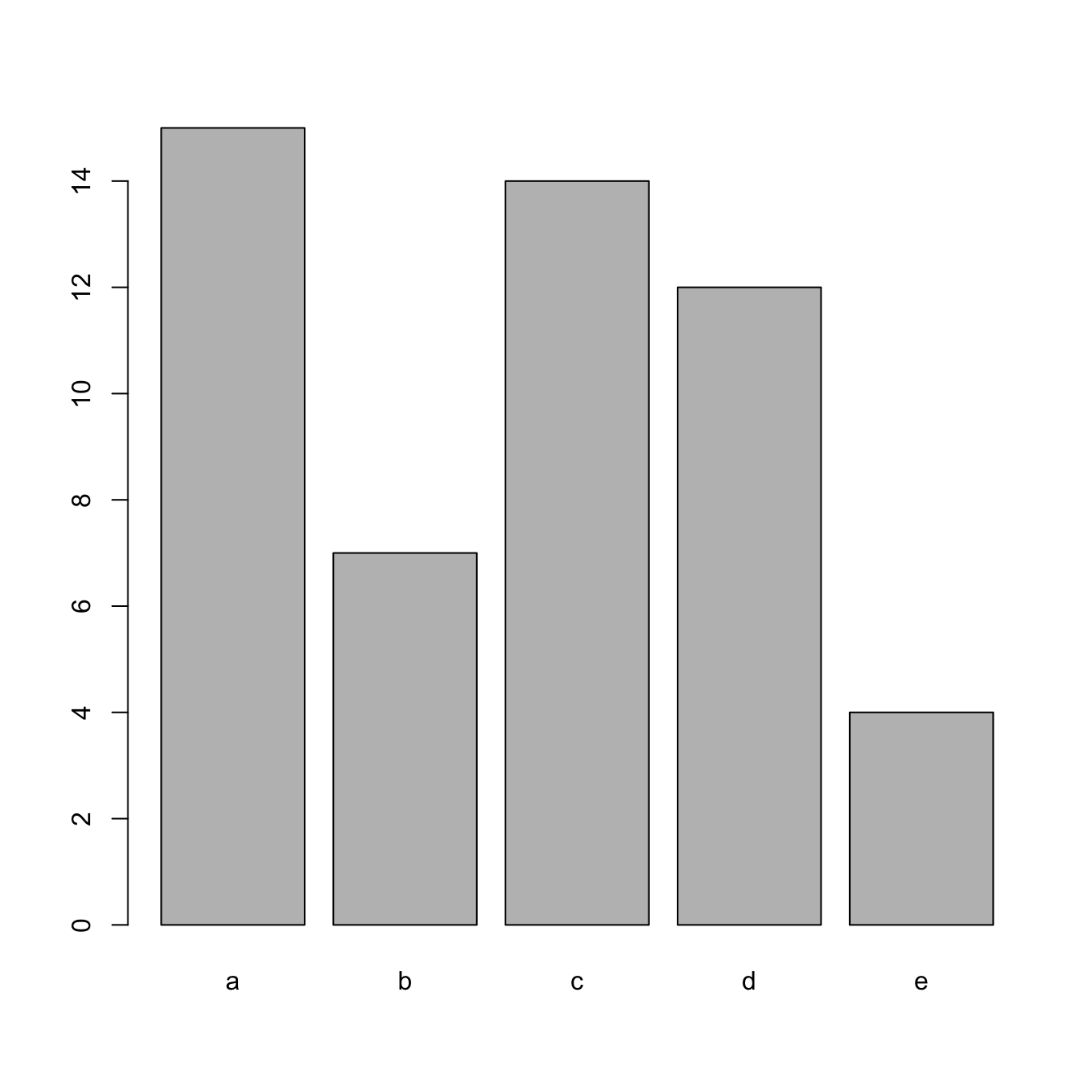``````# create dummy data
data <- data.frame(
name=letters[1:5],
value=sample(seq(4,15),5)
)

# The most basic barplot you can do:
barplot(height=data\$value, names=data\$name)``````

# Custom color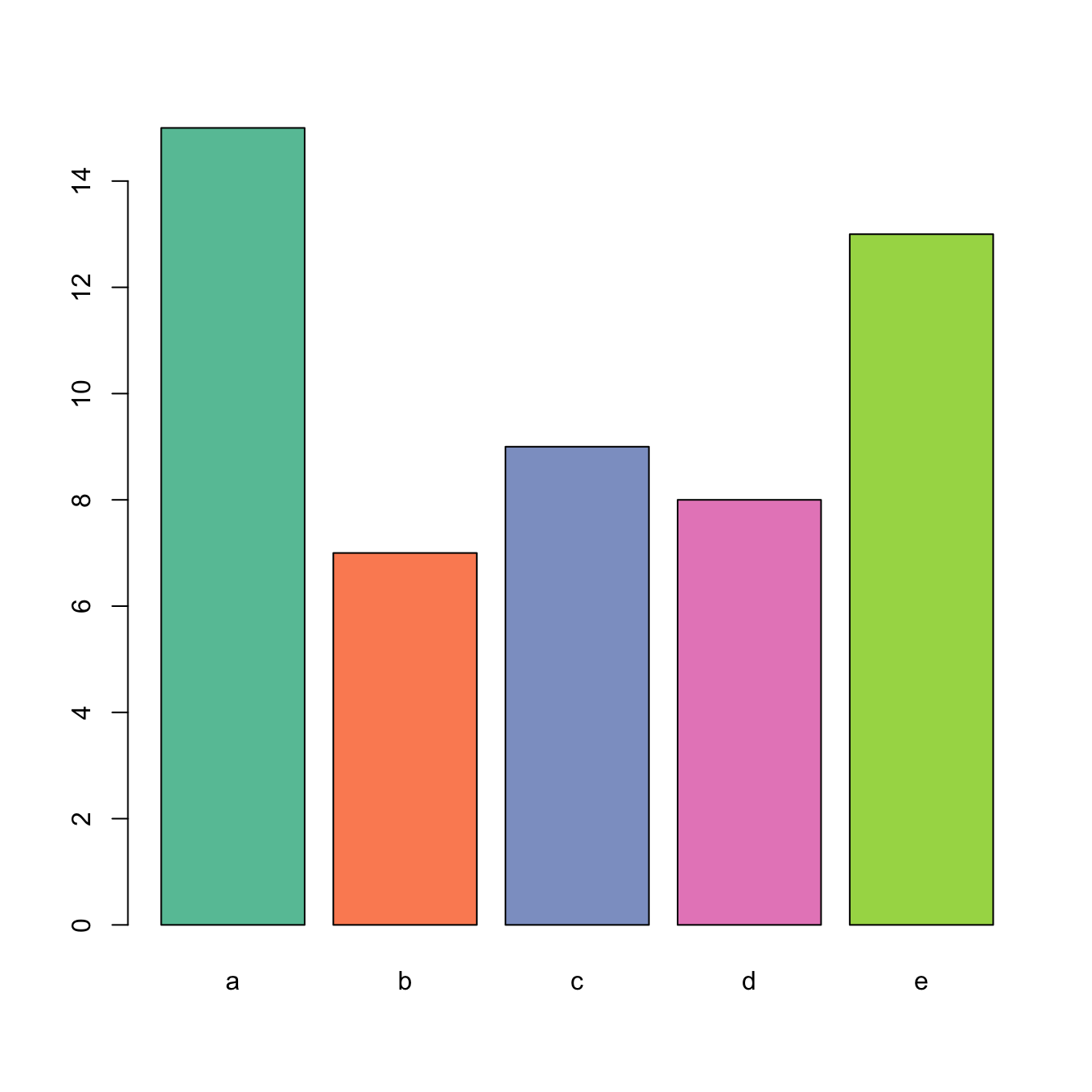Here are 2 examples showing how to custom the barplot color:

• uniform color with `col`, asking one color only
• using a palette coming from `RColorBrewer`
• change border color with the `border` argument
``````# create dummy data
data <- data.frame(
name=letters[1:5],
value=sample(seq(4,15),5)
)

# Uniform color
barplot(height=data\$value, names=data\$name, col=rgb(0.2,0.4,0.6,0.6) )

# Specific color for each bar? Use a well known palette
library(RColorBrewer)
coul <- brewer.pal(5, "Set2")
barplot(height=data\$value, names=data\$name, col=coul )

# Change border color
barplot(height=data\$value, names=data\$name, border="#69b3a2", col="white" )``````

# Title, Axis label, Custom limits

Usual customizations with `xlab`, `ylab`, `main` and `ylim`.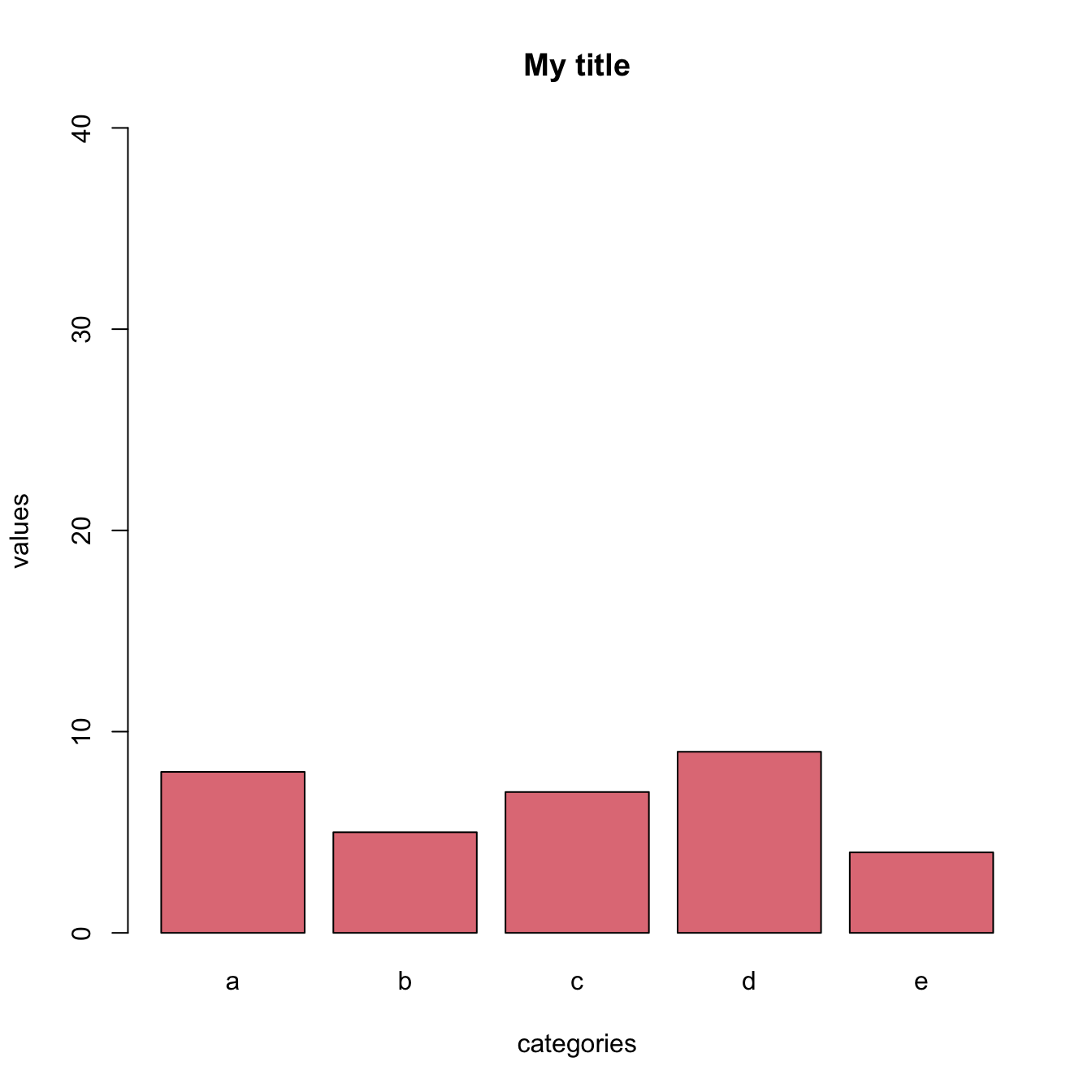``````# create dummy data
data <- data.frame(
name=letters[1:5],
value=sample(seq(4,15),5)
)

# Uniform color
barplot(height=data\$value, names=data\$name,
col=rgb(0.8,0.1,0.1,0.6),
xlab="categories",
ylab="values",
main="My title",
ylim=c(0,40)
)``````

# Horizontal barplot

Usual customizations with `xlab`, `ylab`, `main` and `ylim`.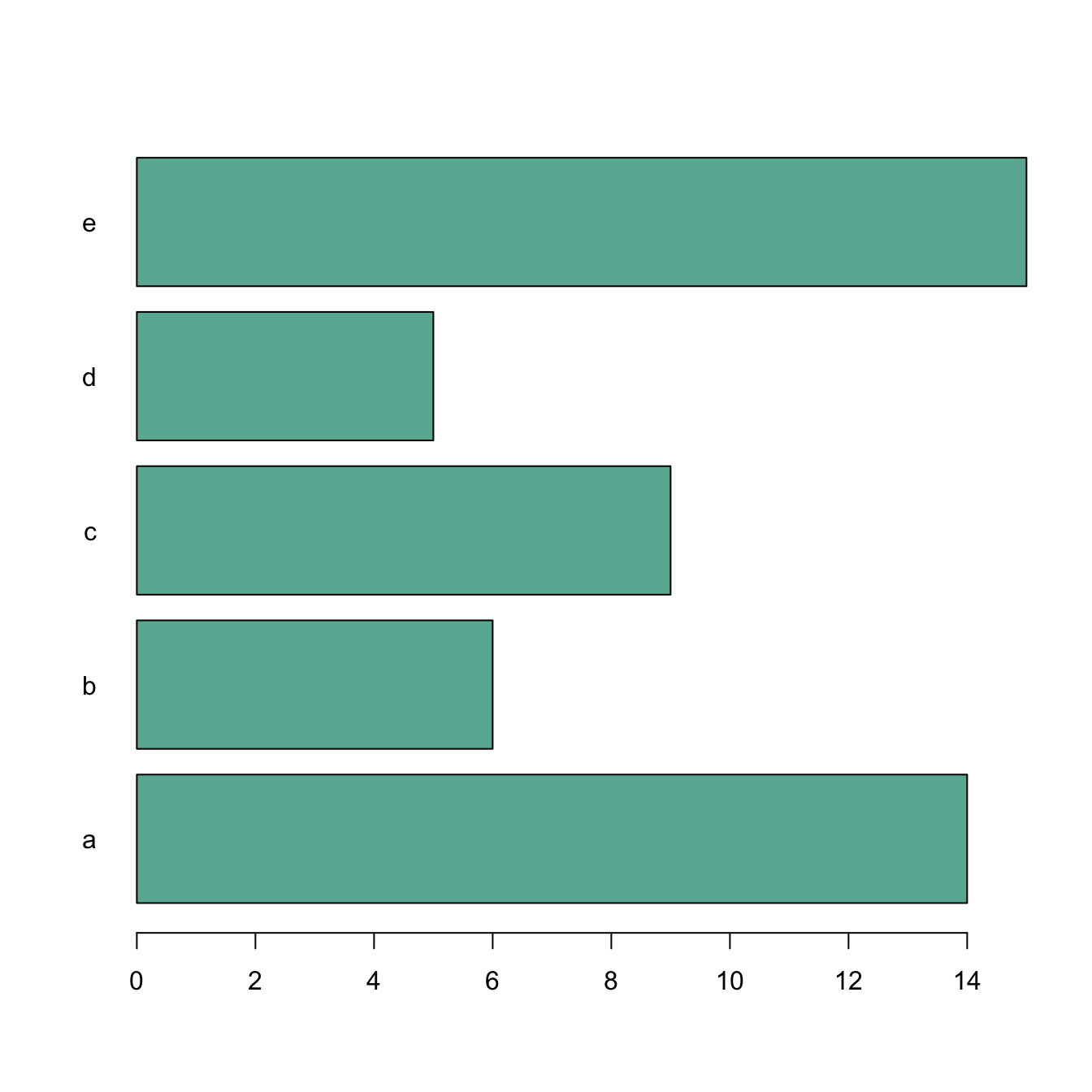``````# create dummy data
data <- data.frame(
name=letters[1:5],
value=sample(seq(4,15),5)
)

# Uniform color
barplot(height=data\$value, names=data\$name,
col="#69b3a2",
horiz=T, las=1
)``````

# Bar width & space between barsIt is possible to control the space between bars and the width of the bars using `space` and `width`.

Can be usefull to represent the number of value behind each bar.

``````# create dummy data
data <- data.frame(
name=letters[1:5],
value=sample(seq(4,15),5)
)

# Control space:
barplot(height=data\$value, names=data\$name, col=rgb(0.2,0.4,0.6,0.6), space=c(0.1,0.2,3,1.5,0.3) )

# Control width:
barplot(height=data\$value, names=data\$name, col=rgb(0.2,0.4,0.6,0.6), width=c(0.1,0.2,3,1.5,0.3) )``````

# Barplot texture

Change bar texture with the `density` and `angle` arguments.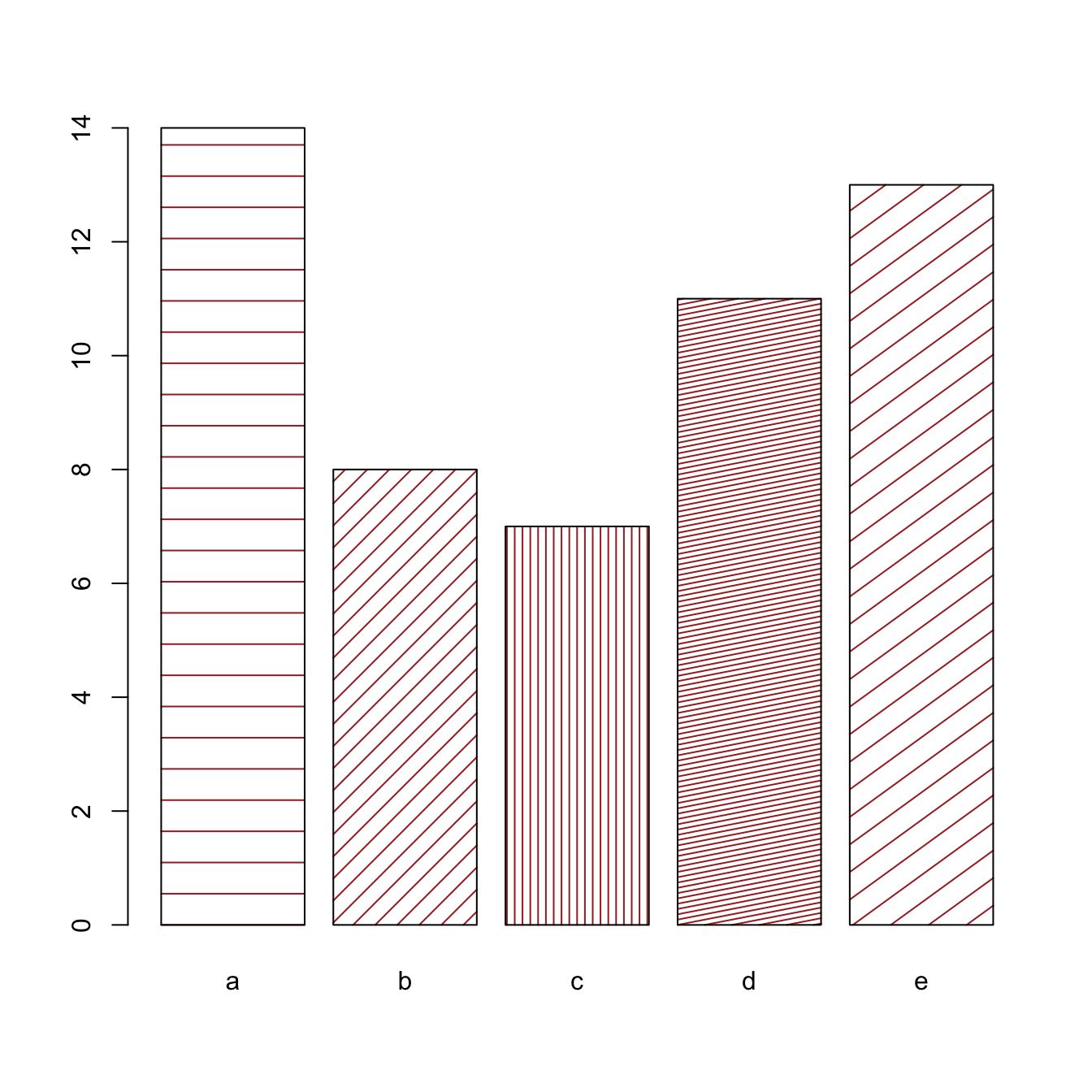``````# create dummy data
data <- data.frame(
name=letters[1:5],
value=sample(seq(4,15),5)
)

# barplot
barplot( height=data\$value, names=data\$name , density=c(5,10,20,30,7) , angle=c(0,45,90,11,36) , col="brown"   )``````

Related chart types

## Contact

This document is a work by Yan Holtz. Any feedback is highly encouraged. You can fill an issue on Github, drop me a message on Twitter, or send an email pasting yan.holtz.data with gmail.com.